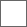• 18回复贴，共1

### 【评分楼】2019年第八届MW杯小组赛第二题评分楼

A.设计分

B.游戏性分

C.加分与扣分

A.设计分

B.游戏性分

C.加分与扣分

A1选手 koopa4 小组赛第二题关卡最终得分：（71.3+72.6）/2=71.95≈72

A.设计分

B.游戏性分

C.加分与扣分

A.设计分

B.游戏性分

C.加分与扣分

A4选手 肥羊羊98 小组赛第二题关卡最终得分：（51.3+54.5）/2=52.9

A.设计分

B.游戏性分

C.加分与扣分

A.设计分

B.游戏性分

C.加分与扣分

C1选手 123568024 小组赛第二题关卡最终得分：（69+70.1）/2=69.55≈69.6

A.设计分

B.游戏性分

C.加分与扣分

A.设计分

B.游戏性分

C.加分与扣分

C2选手 把僵尸炖了 小组赛第二题关卡最终得分：（88.5+89.6）/2=89.05≈89.1

A.设计分

B.游戏性分

C.加分与扣分

A.设计分

B.游戏性分

C.加分与扣分

D3选手 小皓宇Tom 小组赛第二题关卡最终得分：（77+83）/2=80

A.设计分

B.游戏性分

C.加分与扣分

A.设计分

B.游戏性分

C.加分与扣分

D1选手 878yfy 小组赛第二题关卡最终得分：（79.8+84）/2=81.9A.设计分

B.游戏性分

C.加分与扣分

A.设计分

B.游戏性分

C.加分与扣分

A3选手 王一凡01234567 小组赛第二题关卡最终得分：（70.3+78.6）/2=74.45≈74.5

A.设计分

B.游戏性分

C.加分与扣分

A.设计分

B.游戏性分

C.加分与扣分

A2选手 2333ty 小组赛第二题关卡最终得分：（89.1+84.9）/2=87

A.设计分

B.游戏性分

C.加分与扣分

A.设计分

B.游戏性分

C.加分与扣分

B1选手 1168438795 小组赛第二题关卡最终得分：（80+76.2）/2=78.1A.设计分

B.游戏性分

C.加分与扣分

A.设计分

B.游戏性分

C.加分与扣分

B4选手 数字1528君 小组赛第二题关卡最终得分：（91.5+90.8）/2=91.15≈91.2A.设计分

B.游戏性分

C.加分与扣分

A.设计分

B.游戏性分

C.加分与扣分

B3选手 我懂你不懂的lz 小组赛第二题关卡最终得分：（71+77.6）/2=74.3

A.设计分

B.游戏性分

C.加分与扣分

A.设计分

B.游戏性分

C.加分与扣分

B2选手 s小s飞s侠s 小组赛第二题参赛关卡最终得分：（89.6+90.9）/2=90.25≈90.3

#### 扫二维码下载贴吧客户端